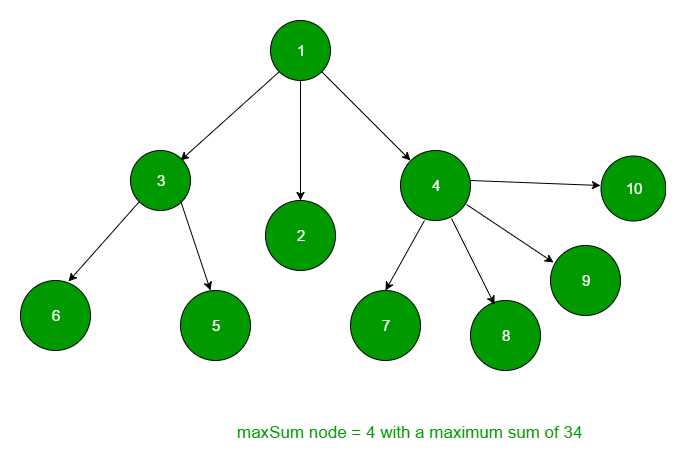Related Articles

# Node having maximum sum of immediate children and itself in n-ary tree

• Difficulty Level : Medium
• Last Updated : 28 Jun, 2021

Given an N-Ary tree, find and return the node for which sum of data of all children and the node itself is maximum. In the sum, data of node itself and data of its immediate children is to be taken.
For example in the given tree,maxSum Node = 4 with maximum sum of 28

The idea is we will maintain a integer variable maxsum which contains the maximum sum yet, and a resnode node pointer which points to the node with maximum sum.
Traverse the tree and maintain the sum of root and data of all its immediate children in currsum
integer variable and update the maxsum variable accordingly.

## C++

 `// CPP program to find the node whose children``// and node sum is maximum.``#include ``using` `namespace` `std;` `// Structure of a node of an n-ary tree``struct` `Node {``    ``int` `key;``    ``vector child;``};` `// Utility function to create a new tree node``Node* newNode(``int` `key)``{``    ``Node* temp = ``new` `Node;``    ``temp->key = key;``    ``return` `temp;``}` `// Helper function to find the node``void` `maxSumUtil(Node* root, Node** resNode,``                ``int``* maxsum)``{``    ``// Base Case``    ``if` `(root == NULL)``        ``return``;` `    ``// curr contains the sum of the root and``    ``// its children``    ``int` `currsum = root->key;` `    ``// total no of children``    ``int` `count = root->child.size();` `    ``// for every child call recursively``    ``for` `(``int` `i = 0; i < count; i++) {``        ``currsum += root->child[i]->key;``        ``maxSumUtil(root->child[i], resNode, maxsum);``    ``}` `    ``// if curr is greater than sum, update it``    ``if` `(currsum > *maxsum) {` `        ``// resultant node``        ``*resNode = root;``        ``*maxsum = currsum;``    ``}``    ``return``;``}` `// Function to find the node having max sum of``// children and node``int` `maxSum(Node* root)``{``    ``// resultant node with max sum of children``    ``// and node``    ``Node* resNode;` `    ``// sum of node and its children``    ``int` `maxsum = 0;` `    ``maxSumUtil(root, &resNode, &maxsum);` `    ``// return the key of resultant node``    ``return` `resNode->key;``}` `// Driver program``int` `main()``{``    ``/*   Let us create below tree``    ``*              1``    ``*          /   |  \``    ``*         2   3   4``    ``*        / \    / |  \ \``    ``*       5   6  7  8  9  10``    ``*/` `    ``Node* root = newNode(1);``    ``(root->child).push_back(newNode(2));``    ``(root->child).push_back(newNode(3));``    ``(root->child).push_back(newNode(4));``    ``(root->child->child).push_back(newNode(5));``    ``(root->child->child).push_back(newNode(6));``    ``(root->child->child).push_back(newNode(5));``    ``(root->child->child).push_back(newNode(6));``    ``(root->child->child).push_back(newNode(6));` `    ``cout << maxSum(root) << endl;` `    ``return` `0;``}`

## Java

 `// Java program to find the node whose children``// and node sum is maximum.``import` `java.util.*;` `class` `GFG``{``    ` `// Structure of a node of an n-ary tree``static` `class` `Node``{``    ``int` `key;``    ``Vector child;``    ``Node()``    ``{``        ``child = ``new` `Vector();``    ``}``};` `// Utility function to create a new tree node``static` `Node newNode(``int` `key)``{``    ``Node temp = ``new` `Node();``    ``temp.key = key;``    ``return` `temp;``}` `static` `int` `maxsum;` `// resultant node with max sum of children``// and node``static` `Node resNode;` `// Helper function to find the node``static` `void` `maxSumUtil(Node root)``{``    ``// Base Case``    ``if` `(root == ``null``)``        ``return``;` `    ``// curr contains the sum of the root and``    ``// its children``    ``int` `currsum = root.key;` `    ``// total no of children``    ``int` `count = root.child.size();` `    ``// for every child call recursively``    ``for` `(``int` `i = ``0``; i < count; i++)``    ``{``        ``currsum += root.child.get(i).key;``        ``maxSumUtil(root.child.get(i));``    ``}` `    ``// if curr is greater than sum, update it``    ``if` `(currsum > maxsum)``    ``{` `        ``// resultant node``        ``resNode = root;``        ``maxsum = currsum;``    ``}``    ``return``;``}` `// Function to find the node having max sum of``// children and node``static` `int` `maxSum(Node root)``{``    ` `    ``// sum of node and its children``    ``int` `maxsum = ``0``;` `    ``maxSumUtil(root);` `    ``// return the key of resultant node``    ``return` `resNode.key;``}` `// Driver code``public` `static` `void` `main(String args[])``{``    ``/* Let us create below tree``                ``1``            ``/ | \``            ``2 3 4``        ``/ \ / | \ \``        ``5 6 7 8 9 10``    ``*/` `    ``Node root = newNode(``1``);``    ``(root.child).add(newNode(``2``));``    ``(root.child).add(newNode(``3``));``    ``(root.child).add(newNode(``4``));``    ``(root.child.get(``0``).child).add(newNode(``5``));``    ``(root.child.get(``0``).child).add(newNode(``6``));``    ``(root.child.get(``2``).child).add(newNode(``5``));``    ``(root.child.get(``2``).child).add(newNode(``6``));``    ``(root.child.get(``2``).child).add(newNode(``6``));` `    ``System.out.print( maxSum(root) );``}``}` `// This code is contributed by Arnab Kundu`

## Python3

 `# Python3 program to find the node``# whose children and node sum is maximum.` `# Structure of a node of an n-ary tree``class` `Node:``    ` `    ``def` `__init__(``self``, key):``        ``self``.key ``=` `key``        ``self``.child ``=` `[]` `# Helper function to find the node``def` `maxSumUtil(root, resNode, maxsum):` `    ``# Base Case``    ``if` `root ``=``=` `None``:``        ``return` `    ``# curr contains the sum of the root``    ``# and its children``    ``currsum ``=` `root.key` `    ``# total no of children``    ``count ``=` `len``(root.child)` `    ``# for every child call recursively``    ``for` `i ``in` `range``(``0``, count):``        ``currsum ``+``=` `root.child[i].key``        ``resNode, maxsum ``=` `maxSumUtil(root.child[i],``                                     ``resNode, maxsum)` `    ``# if curr is greater than sum,``    ``# update it``    ``if` `currsum > maxsum:` `        ``# resultant node``        ``resNode ``=` `root``        ``maxsum ``=` `currsum``    ` `    ``return` `resNode, maxsum` `# Function to find the node having``# max sum of children and node``def` `maxSum(root):` `    ``# resultant node with max``    ``# sum of children and node``    ``resNode, maxsum ``=` `Node(``None``), ``0``    ``resNode, maxsum ``=` `maxSumUtil(root, resNode,``                                       ``maxsum)` `    ``# return the key of resultant node``    ``return` `resNode.key` `# Driver Code``if` `__name__ ``=``=` `"__main__"``:` `    ``root ``=` `Node(``1``)``    ``(root.child).append(Node(``2``))``    ``(root.child).append(Node(``3``))``    ``(root.child).append(Node(``4``))``    ``(root.child[``0``].child).append(Node(``5``))``    ``(root.child[``0``].child).append(Node(``6``))``    ``(root.child[``2``].child).append(Node(``5``))``    ``(root.child[``2``].child).append(Node(``6``))``    ``(root.child[``2``].child).append(Node(``6``))` `    ``print``(maxSum(root))` `# This code is contributed by Rituraj Jain`

## C#

 `// C# program to find the node whose children``// and node sum is maximum``using` `System;``using` `System.Collections.Generic;` `class` `GFG``{``    ` `// Structure of a node of an n-ary tree``public` `class` `Node``{``    ``public` `int` `key;``    ``public` `List child;``    ``public` `Node()``    ``{``        ``child = ``new` `List();``    ``}``};` `// Utility function to create a new tree node``static` `Node newNode(``int` `key)``{``    ``Node temp = ``new` `Node();``    ``temp.key = key;``    ``return` `temp;``}` `static` `int` `maxsum;` `// resultant node with max sum of children``// and node``static` `Node resNode;` `// Helper function to find the node``static` `void` `maxSumUtil(Node root)``{``    ``// Base Case``    ``if` `(root == ``null``)``        ``return``;` `    ``// curr contains the sum of the root and``    ``// its children``    ``int` `currsum = root.key;` `    ``// total no of children``    ``int` `count = root.child.Count;` `    ``// for every child call recursively``    ``for` `(``int` `i = 0; i < count; i++)``    ``{``        ``currsum += root.child[i].key;``        ``maxSumUtil(root.child[i]);``    ``}` `    ``// if curr is greater than sum, update it``    ``if` `(currsum > maxsum)``    ``{` `        ``// resultant node``        ``resNode = root;``        ``maxsum = currsum;``    ``}``    ``return``;``}` `// Function to find the node having max sum of``// children and node``static` `int` `maxSum(Node root)``{``    ` `    ``// sum of node and its children``    ``int` `maxsum = 0;` `    ``maxSumUtil(root);` `    ``// return the key of resultant node``    ``return` `resNode.key;``}` `// Driver code``public` `static` `void` `Main(String []args)``{``    ``/* Let us create below tree``                ``1``            ``/ | \``            ``2 3 4``        ``/ \ / | \ \``        ``5 6 7 8 9 10``    ``*/` `    ``Node root = newNode(1);``    ``(root.child).Add(newNode(2));``    ``(root.child).Add(newNode(3));``    ``(root.child).Add(newNode(4));``    ``(root.child.child).Add(newNode(5));``    ``(root.child.child).Add(newNode(6));``    ``(root.child.child).Add(newNode(5));``    ``(root.child.child).Add(newNode(6));``    ``(root.child.child).Add(newNode(6));` `    ``Console.Write( maxSum(root) );``}``}` `// This code has been contributed by 29AjayKumar`

## Javascript

 ``

Output:

`4`

This article is contributed by Chhavi. If you like GeeksforGeeks and would like to contribute, you can also write an article using write.geeksforgeeks.org or mail your article to review-team@geeksforgeeks.org. See your article appearing on the GeeksforGeeks main page and help other Geeks.